# TS系列篇｜高级数据类型

"不畏惧，不将就，未来的日子好好努力"——大家好！我是小芝麻😄

## 1、交叉类型`&`

• 交叉类型是将多个类型合并为一个类型。交叉类型其实就是两个接口类型的属性的并集
``````type PersonName = { name: string }
type Person = PersonName & { age: number }

let person: Person = {
name: '金色小芝麻',
age: 18
}

• 在合并多个类型的过程中，刚好出现某些类型存在相同的成员，但对应的类型又不一致时
``````interface X {
c: string;
d: string;
}

interface Y {
c: number;
e: string
}

type XY = X & Y;
type YX = Y & X;

let p: XY = { c: 6, d: "d", e: "e" }; // ERROR 不能将类型“number”分配给类型“never”。
let q: YX = { c: "c", d: "d", e: "e" }; // ERROR 不能将类型“string”分配给类型“never”。

## 2、联合类型 `|`

• 联合类型（Union Types）表示取值可以为多种类型中的一种
• 未赋值时联合类型上只能访问两种类型共有的属性和方法
``````let name: string | number
name = 3
name = '金色小芝麻'

``````name = true // ERROR 不能将类型“true”分配给类型“string | number”。

### 访问联合类型的属性或方法

``````function getLength(something: string | number): number {
return something.length; // ERROR
}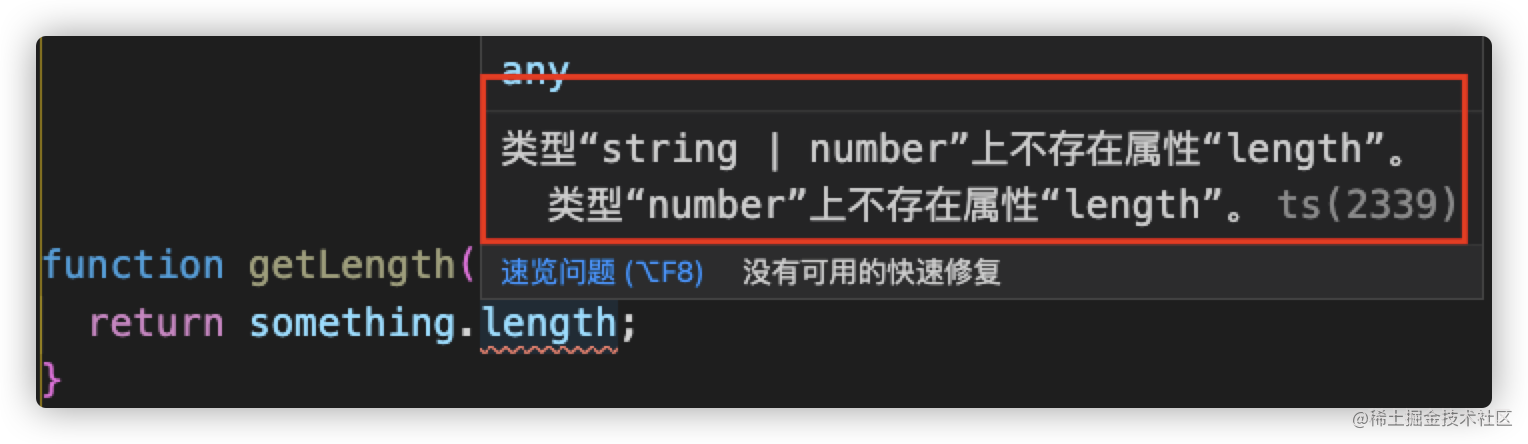上例中，`length` 不是 `string` 和 `number` 的共有属性，所以会报错。

``````function getString(something: string | number): string {
return something.toString();
}

``````let name: string | number
name = 3
console.log(name.length) // ERROR 类型“number”上不存在属性“length”。
name = '金色小芝麻'
console.log(name.length) // 5

## 3、字面量类型

• 可以把字符串、数字、布尔值字面量组成一个联合类型
``````type ZType = 1 | 'one' | true
let t1: ZType = 1
let t2: ZType = 'one'
let t3: ZType = true

// 字面量类型
let Gender3: 'BOY' | 'GIRL'
Gender3 = 'BOY' // 可以编译
Gender3 = 'GIRL' // 可以编译
Gender3 = true // 编译失败

### 3.1 字符串字面量类型

``````type Direction = 'North' | 'East' | 'South' | 'West';
function move(distance: number, direction: Direction) {
// ...
}

move(1, 'North');  // YES
move(1, '小芝麻'); // ERROR，类型“"小芝麻"”的参数不能赋给类型“Direction”的参数。

### 字符串字面量 VS 联合类型

• 字符串字面量类型用来约束取值只能是某`几个字符串`中的一个，联合类型表示取值可以为`多种类型`中的一种
• 字符串字面量 限定了使用该字面量的地方仅接受特定的值，联合类型 对于值并没有限定，仅仅限定值的类型需要保持一致

### 3.2 数字字面量类型

``````type Direction = 11 | 12 | 13
function move(distance: number, direction: Direction) {
// ...
}

move(1, 11);  // YES
move(1, 1); // ERROR，类型“1”的参数不能赋给类型“Direction”的参数。

## 4、索引类型 `keyof`

• 使用索引类型，编译器就能够检查使用了动态属性名的代码。

``````let obj = {
a: 1,
b: 2,
c: 3
}

function getValues(obj: any, keys: string[]) {
return keys.map(key => obj[key])
}

console.log(getValues(obj, ['a', 'b'])) // [ 1, 2 ]

console.log(getValues(obj, ['a', 'f'])) // [ 1, undefined ]

• 索引类型的查询操作符：`keyof T` (表示类型 T 的所有公共属性的字面量的联合类型)
• 索引访问操作符: `T[K]`
• 泛型约束: `T extends U`

• 首先我们需要一个泛型 `T` 它来代表传入的参数 `obj` 的类型，因为我们在编写代码时无法确定参数 `obj` 的类型到底是什么，所以在这种情况下要获取 `obj` 的类型必须用面向未来的类型--泛型。
``````function getValues< T >(obj: T, keys: string[]) {
return keys.map(key => obj[key])
}

• 那么传入的第二个参数 `keys` ，它的特点就是数组的成员必须由参数 `obj` 的属性名称构成，这个时候我们很容易想到刚学习的操作符`keyof``keyof T`代表参数 `obj` 类型的属性名的联合类型，我们的参数`keys` 的成员类型`K`则只需要约束到`keyof T`即可。
``````function getValues<T, K extends keyof T>(obj: T, keys: K[]) {
return keys.map(key => obj[key])
}

• 返回值就是，我们通过类型访问符`T[K]`便可以取得对应属性值的类型，他们的数组`T[K][]`正是返回值的类型。
``````function getValues<T, K extends keyof T>(obj: T, keys: K[]): T[K][] {
return keys.map(key => obj[key])
}

``````console.log(getValues(obj, ['a', 'b'])) // [ 1, 2 ]
console.log(getValues(obj, ['a', 'f'])) // ERROR 不能将类型“"f"”分配给类型“"a" | "b" | "c"”。

## 5、映射类型 `in`

``````interface Person {
name: string
age: number
gender: 'male' | 'female'
}

• K：类型变量，依次绑定到每个属性上，对应每个属性名的类型
• Keys：字符串字面量构成的联合类型，表示一组属性名（的类型）

• 首先，我们得找到`Keys`，即字符串字面量构成的联合类型，这就得使用上面提到的`keyof`操作符，我们传入的类型是`Person`，得到`keyof Person`，即传入类型`Person`的属性名的联合类型。

• 然后我们需要将`keyof Person`的属性名称一一映射出来`[key in keyof Person]`，如果我们要把所有的属性成员变为可选类型，那么需要`Person[key]`取出相应的属性值，最后我们重新生成一个可选的新类型`{ [key in keyof Person]?: Person[key] }`

``````type PartPerson = {
[key in keyof Person]?: Person[key]
}
let p1: PartPerson = {}
// 也可以使用泛型
type Part<T> = {
[key in keyof T]?: T[key]
}
let p2: Part<Person> = {}

### 5.1 内置工具映射类型

• TS 中内置了一些工具类型来帮助我们更好地使用类型系统（可以在 lib.es5.d.ts 中查看实现原理）
``````interface obj {
a: string;
b: number;
c: boolean;
}

#### 5.1.1 Partial

• Partial<T> 可以将传入的属性由非可选变为可选返回：
``````type PartialObj = Partial<obj>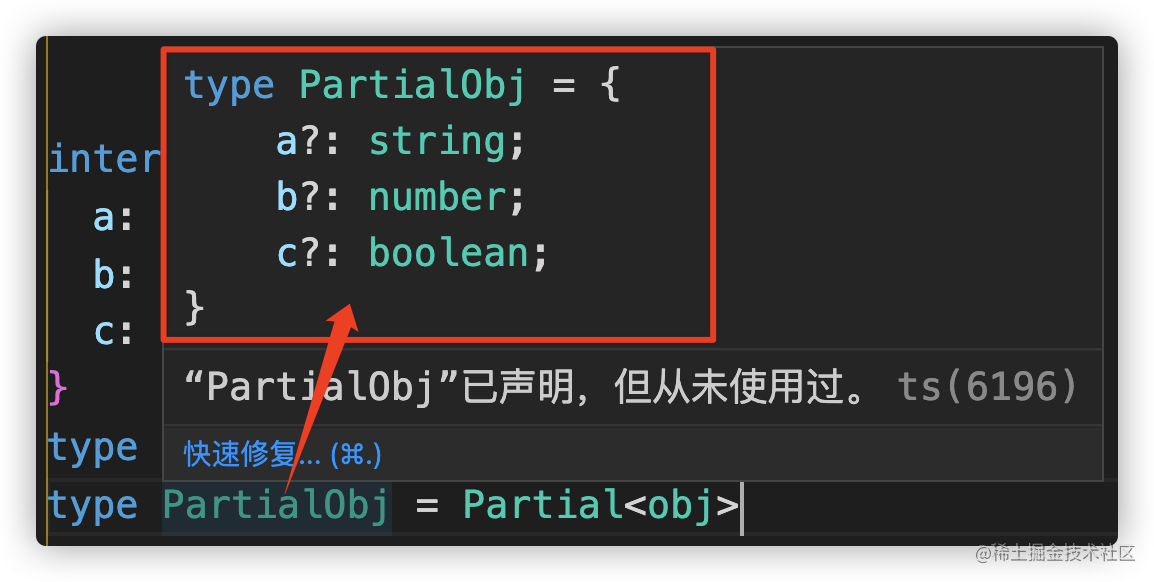#### 5.1.2 Required

• Required<T> 可以将传入的属性变为必选返回：
``````type RequiredObj = Required<PartialObj>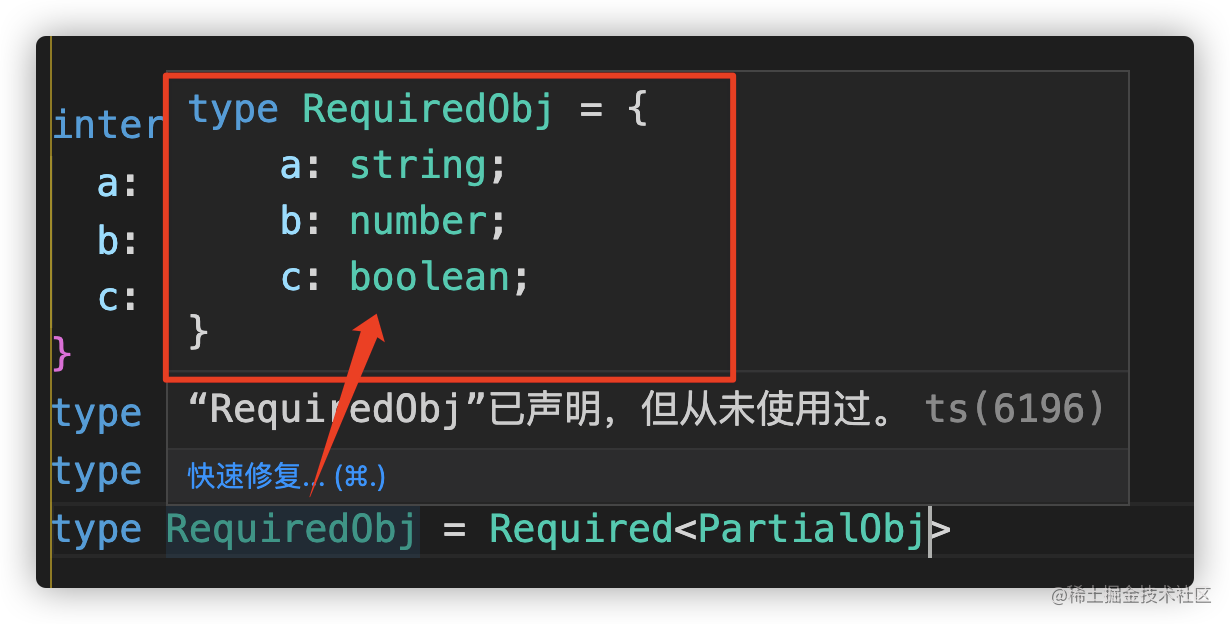``````type ReadonlyObj = Readonly<obj>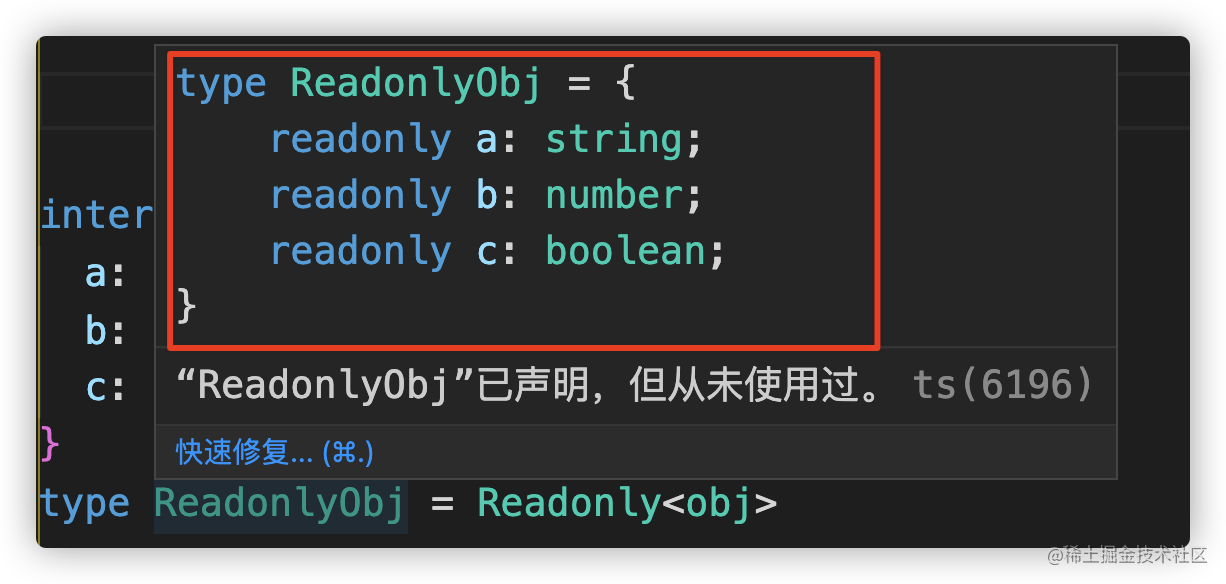#### 5.1.4 Pick

• Pick<T, K extends keyof T> 能够帮助我们从传入的属性中摘取某项返回
``````type PickObj = Pick<obj, 'a' | 'b'>
// 从 obj 中 摘取 a 和 b 属性返回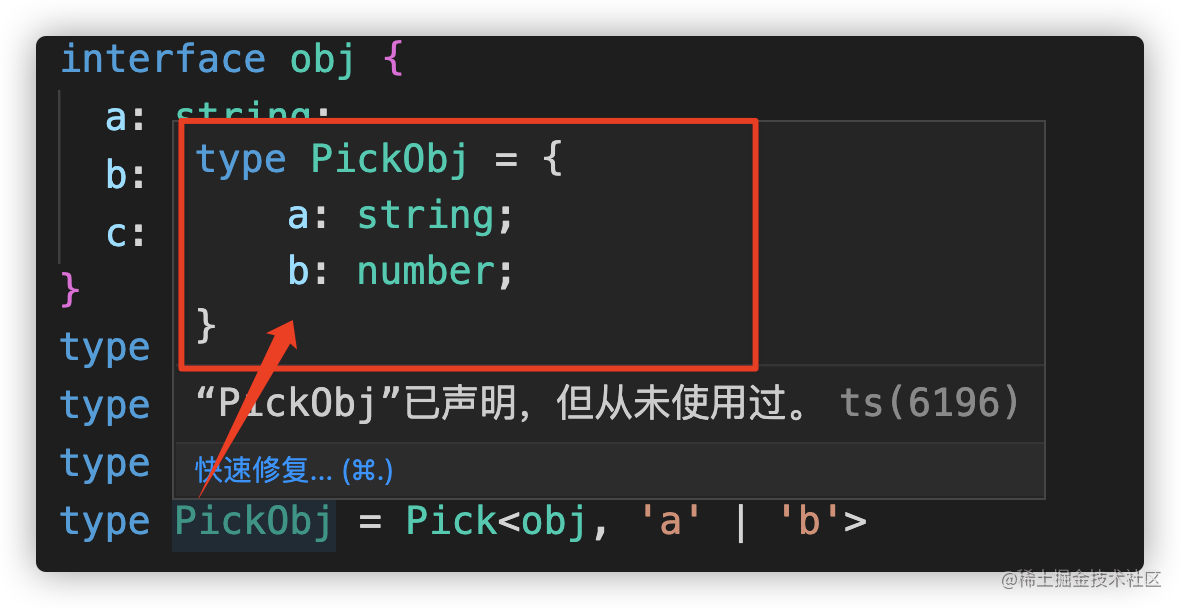``````interface Animal {
name: string
age: number
}
// 摘取 Animal 中的 name 属性
type AnimalSub = Pick<Animal, 'name'> // {name: string}
let a: AnimalSub = { name: '金色小芝麻' }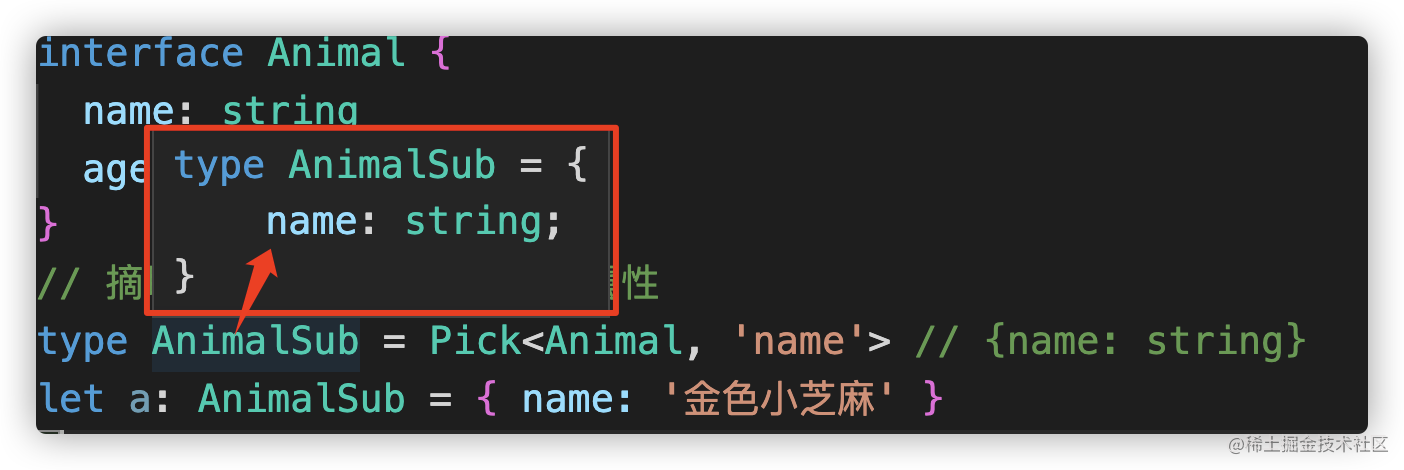#### 5.1.5 Record

• Record<T, U> 将字符串字面量类型 `T` 中所有字串变量作为新类型的 `Key` 值， `U` 类型作为 `Key` 的类型
``````type RecordObj = Record<'x' | 'y', obj>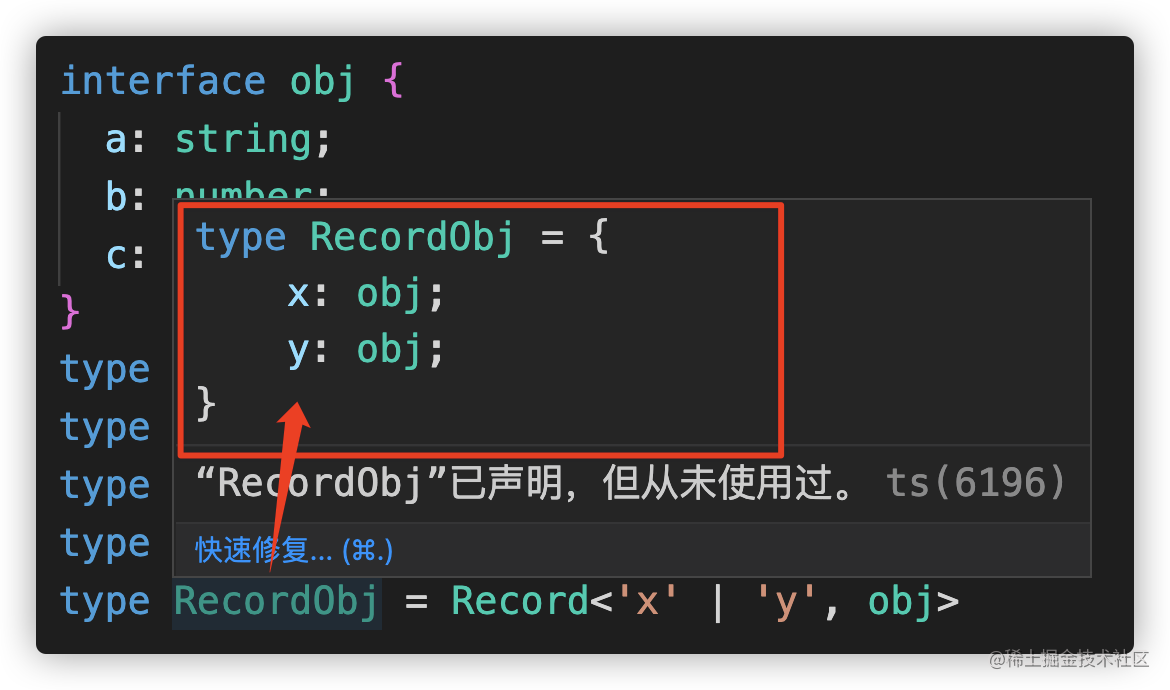### 5.2 映射类型修饰符的控制

• TypeScript 2.8 中增加了对映射类型修饰符的控制
• 具体而言，一个 `readonly``?`修饰符在一个映射类型里可以用前缀 `+``-`来表示这个修饰符应该被添加或删除
• TS 中部分内置工具类型就利用了这个特性（Partial、Required、Readonly...）, 这里我们可以参考 Partial、Required 的实现
``````type MutableRequired<T> = { -readonly [P in keyof T]-?: T[P] }; // 移除readonly和?

``````type ReadonlyPartial<T> = { readonly [P in keyof T]?: T[P] }; // 添加readonly和?

## 6、条件类型

TypeScript 2.8引入了条件类型，它能够表示非统一的类型。 条件类型会以一个条件表达式进行类型关系检测，从而在两种类型中选择其一：

``````T extends U ? X : Y

### 6.1 分布式条件类型

``````A|B|C extends U ? X : Y
// 等价于
A extends U ? X : Y | B extends U ? X : Y | C extends U ? X : Y

``````interface Person {
name: string;
age: number;
getName(): string;
}
declare function add\<T>(x: T): T extends Person ? string : number;
function func\<U>(x: U) {
let b: string | number = a;
}

• 分布式条件类型可以用来过滤联合类型，如下所示，Filter<T, U>类型可从T中移除U的子类型。
``````type Filter<T, U> = T extends U ? never : T;
type T1 = Filter<"a" | "b" | "c" | "d", "a" | "c" | "f">; // "b" | "d"
type T2 = Filter<string | number | (() => void), Function>; // string | number

• 分布式条件类型也可与映射类型配合使用，进行针对性的类型映射，即不同源类型对应不同映射规则，例如映射接口的方法名，如下所示。
``````interface Person {
name: string;
age: number;
getName(): string;
}

type FunctionPropertyNames<T> = {
[K in keyof T]: T[K] extends Function ? K : never
}[keyof T];
type T3 = FunctionPropertyNames<Person>; // "getName"

### 6.2 类型推断 `infer`

``````type Func<T> = T extends (...args: any[]) => infer R ? R : any;

• 当函数具有重载时，就取最后一个函数签名进行推断，如下所示，其中ReturnType<T>是内置的条件类型，可获取函数类型T的返回值类型。
``````declare function load(x: string): number;
declare function load(x: string | number): string | number;
type T4 = ReturnType<typeof load>; // string | number

• 注意，无法在正常类型参数的约束子语句中使用infer声明，如下所示。
``````type Func<T extends (...args: any[]) => infer R> = R; // 错误，不支持

• 但是可以将约束里的类型变量移除，并将其转移到条件类型中，就能达到相同的效果，如下所示。
``````type AnyFunction = (...args: any[]) => any;
type Func<T extends AnyFunction> = T extends (...args: any[]) => infer R ? R : any;

### 6.3 预定义条件类型

TypeScript 2.8在`lib.d.ts`里增加了一些预定义的条件类型(可以在 lib.es5.d.ts 中查看；)：

• 1）Exclude<T, U>：从T中移除掉U的子类型。
• 2）Extract<T, U>：从T中筛选出U的子类型。
• 3）NonNullable<T>：从T中移除null与undefined。
• 4）ReturnType<T>：获取函数返回值类型。
• 5）InstanceType<T>：获取构造函数的实例类型。

#### 6.3.1 Exclude

• 从 T 可分配给的类型中排除 U
``````type E = Exclude<string | number, string>
let e: E = 10 // number

#### 6.3.2 Extract

• 从 T 可分配给的类型中提取 U
``````type E = Extract<string | number, string>
let e: E = '1' // string

#### 6.3.3 NonNullable

• 从 T 中排除 null 和 undefined
``````type E = NonNullable<string | number | null | undefined>
let e: E = '1' // string | number

#### 6.3.4 ReturnType

• 获取函数类型的返回类型
``````function getUserInfo() {
return { name: '金色小芝麻', age: 10 }
}
type UserInfo = ReturnType<typeof getUserInfo>
let user: UserInfo = { name: '金色小芝麻', age: 10 } // {name: string;age: number;}

#### 6.3.5 InstanceType

• 获取构造函数的实例类型
``````class Person {
name: string
constructor(name: string) {
this.name = name
}
}
type P = InstanceType<typeof Person>
let p: P = new Person('1') // Person

## 参考文献

. TypeScript中文网